Courses

# Ex 3.4 NCERT Solutions- Pair of Linear Equations in Two Variables Class 10 Notes | EduRev

## Class 10 : Ex 3.4 NCERT Solutions- Pair of Linear Equations in Two Variables Class 10 Notes | EduRev

The document Ex 3.4 NCERT Solutions- Pair of Linear Equations in Two Variables Class 10 Notes | EduRev is a part of the Class 10 Course Class 10 Mathematics by VP Classes.
All you need of Class 10 at this link: Class 10

Ques 1: Solve the following pair of linear equations by the elimination method and the substitution method:
(i) x + y = 5 and 2x - 3y = 4
(ii) 3x + 4y = 10 and 2x - 2y = 2
(iii) 3x - 5y - 4 = 0 and 9x = 2y + 7
(iv)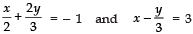Sol: (i) x + y = 5   ...(1)
2x - 3y = 4   ...(2)
Multiplying (1) by 3 and adding it to (2),
3 [x + y = 5] ⇒ 3x + 3y = 15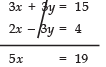⇒ x = (19/5)
Now, putting x = 19/5 in (1), we get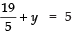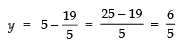Thus, x = 19/5 and y = 6/5
(ii) 3x + 4y = 10  ...(1)
2x - 2y = 2  ...(2)
From equation (2), we have:
2 [2x - 2y = 2] ⇒ 4x - 4y = 4 ...(3)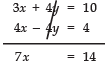⇒ x = 14/7 = 2
Putting x = 2 in (1), we get
3 (2) + 4y = 10
⇒ 6 + 4y = 10
⇒ 4y = 10 - 6 = 4
⇒ y = 4/4 = 1
Thus, x = 2 and y = 1
(iii) 3x - 5y - 4 = 0   ...(1)
9x = 2y + 7 or 9x - 2y - 7 = 0   ...(2)
From equation (1), we have:
3 [3x - 5y - 4 = 0]  ⇒ 9x - 15y - 12 = 0 ...(3)
Subtracting (2) from (3),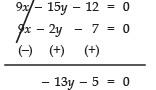⇒ y = (-5/13)
Now from (1), we get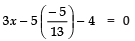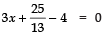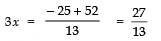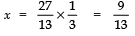Thus, x = 9/13 and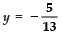(iv)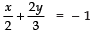...(1)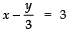..(2)
Multiplying equation (2) by 2,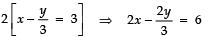Adding (1) and (3), we have: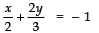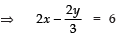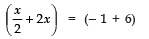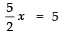⇒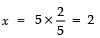Putting x = 2 in (1), we get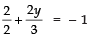⇒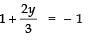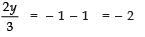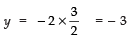Thus, x =2 and y = - 3

Ques 2: Form the pair of linear equations in the following problems, and find their solutions (if they exist) by the elimination method:
(i) If we add 1 to the numerator and subtract 1 from the denominator, a fraction reduces to 1. It becomes 1/2 if we only add 1 to the denominator. What is the fraction?
(ii) Five years ago, Nuri was thrice as old as Sonu. Ten years later, Nuri will be twice as old as Sonu. How old are Nuri and Sonu?
(iii) The sum of the digits of a two-digit number is 9. Also, nine times this number is twice the number obtained by reversing the order of the digits. Find the number.
(iv) Meena went to a bank to withdraw ₹ 2000. She asked the cashier to give her ₹ 50 and ₹ 100 notes only. Meena got 25 notes in all. Find how many notes of ₹ 50 and ₹ 100 she received.
(v) A lending library has a fixed charge for the first three days and an additional charge for each day thereafter. Saritha paid ₹ 27 for a book kept for seven days, while Susy paid ₹ 21 for the book she kept for five days. Find the fixed charge and the charge for each extra day.
Sol: (i) Let the numerator = x
And the denominator = y
∴ Fraction = [x/y]

Case I: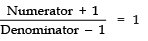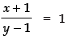⇒ x + 1 = y - 1
x - y = - 1 - 1 = - 2
⇒ x - y = - 2   ...(1)
Case II: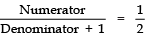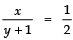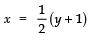...(2)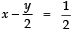Subtracting (3) from (1), we have: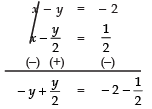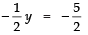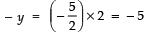⇒ y = 5
Now, putting y = 5 in (2), we have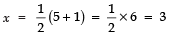Thus x = 3 and y = 5
∴The required fraction = 3/5
(ii) Let the present age of Nuri = x years
And the present age of Sonu = y years.
5 years ago:
Age of Nuri = (x - 5) yrs
Age of Sonu = (y - 5) yrs
Condition:
Age of Nuri = 3 [Age of Sonu]
⇒ x - 5 = 3 [y - 5]
⇒ x - 5 = 3y - 15
⇒ x - 3y - 5 + 15 = 0
⇒ x - 3y + 10 = 0 ...(1)
10 years later:
Age of Nuri = (x + 10) years
Age of Sonu = (y + 10) yrs
Condition:
Age of Nuri = 2 [Age of Sonu]
⇒ x + 10 = 2 (y + 10)
⇒ x + 10 = 2y + 20
⇒ x - 2y + 10 - 20 = 0
⇒ x - 2y - 10 = 0 ...(2)
Subtracting (1) from (2),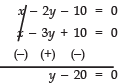⇒ y = 20
Putting y = 20 in (1), we get
x - 3 (20) + 10 = 0
⇒ x - 60 + 10 = 0
⇒ x - 50 = 0 ⇒ x = 50
Thus, x = 50 and y = 20
∴ Age of Nuri = 50 years
Age of Sonu = 20 years
(iii) Let the digit at unit place = x
And the digit at tens place = y
∴ The number = 10y + x
The number obtained by reversing the digits = 10x + y
∵ 9 [The number] = 2 [Number obtained by reversing the digits]
∴ 9 [10y + x] = 2 [10x + y]
⇒ 90y + 9x = 20x + 2y
⇒ 90y + 9x - 20x - 2y = 0
⇒ - 11x + 88y = 0
⇒ x - 8y = 0   ...(1)
Also Sum of the digits = 9
∴ x + y = 9   ...(2)
Subtracting (1) from (2), we have :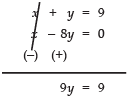⇒ y = 9/9  = 1
Putting y = 1 in (2), we get

x + 1 = 9
⇒ x = 9 - 1 = 8
Thus, x = 8 and y = 1
∴ The required number = (10 × 1) + 8
= 10 + 8
= 18
(iv) Let the number of 50 rupee notes = x
And the number of 100 rupee notes = y
According to the conditions
Total number of notes = 25
∴ x + y = 25    ...(1)
∵ The value of all the notes = ₹ 2000
∴ 50x + 100y = 2000
⇒ x + 2y = 40   ...(2)
Subtracting equation (1) from (2),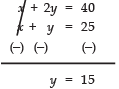Putting y = 15 in (1),
x + 15 = 25
⇒ x = 25 - 15 = 10
Thus, x = 10 and y = 15
⇒ Number of 50 rupee notes = 10
Number of 100 rupee notes = 15
(v) Let the fixed charge (for first three days) = ₹ x
And the additional charge for each extra day = ₹ y
First Condition,
Charge for 7 days = ₹ 27
∴  x + 4y = 27    ...(1)
[∵ Extra days = 7 - 3 = 4]
Charge for 5 days = ₹ 21
⇒ x + 2y = 21  ...(2)
[∵ Extra days = 5 - 3 = 2]
Subtracting (2) from (1), we get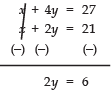⇒ y = 6/2 = 3
Putting y = 3 in (2), we have:

x + 2 (3) = 21
x + 6 = 21
x = 21 - 6 = 15
Since x = 15  and  y = 3
∴Fixed charge = ₹ 15
Additional per day charge = ₹ 3

l Cross-Multiplication Method
In cross-multiplication method, we use the following steps:
I. Write down the given pair of equations in the form of:
a1 x + b1 y + c1 = 0
a2 x + b2 y + c2 = 0
II. Write the coefficients with the column y, constant terms, coefficients of x and repeating with that of y as shown below:
b1  c1  a1  b1
b2  c2  a2  b2
III. Now, write the coefficients of x, y and 1 in the following manner.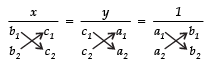Note:
(i) Below x, we write coefficients of y and constant terms.
(ii) Below y, we write constant terms and coefficients of x.
(iii) Below 1, we write coefficients of x and y.

IV. The expressions in the denominator of x, y and 1 are obtained by multiplying the number written at the end of arrows such that:
(i) Numbers with downward arrows are multiplied first.
(ii) Then the products of numbers with upwards arrows are subtracted from the above products.
i.e.,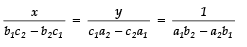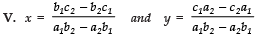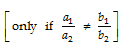Note:
I. The cross-multiplication method can be used only for the consistent systems of linear equations. We cannot use it for the inconsistent and dependent systems.
II. In case of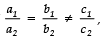the system has no solution because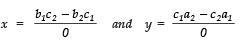and division by zero is not defined.
III. In case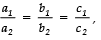, the values of x and y are reduced to  form i.e.,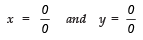Such systems are dependent and pair of given equations have unlimited solutions.

Offer running on EduRev: Apply code STAYHOME200 to get INR 200 off on our premium plan EduRev Infinity!

132 docs

,

,

,

,

,

,

,

,

,

,

,

,

,

,

,

,

,

,

,

,

,

;